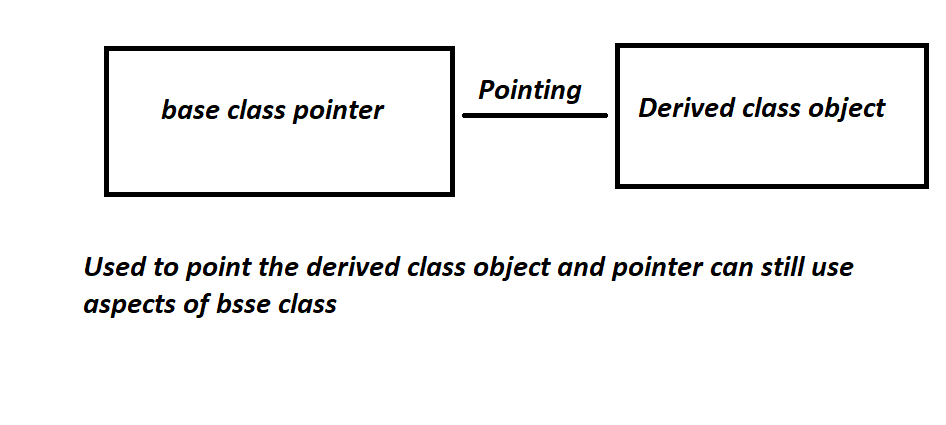Base class pointer pointing to derived class object

• Difficulty Level : Easy
• Last Updated : 23 Aug, 2021

Pointer is a data type that stores the address of other data types.

The pointer of Base Class pointing different object of derived class:Approach:

• A derived class is a class which takes some properties from its base class.
• It is true that a pointer of one class can point to other class, but classes must be a base and derived class, then it is possible.
• To access the variable of the base class, base class pointer will be used.
• So, a pointer is type of base class, and it can access all, public function and variables of base class since pointer is of base class, this is known as binding pointer.
• In this pointer base class is owned by base class but points to derived class object.
• Same works with derived class pointer, values is changed.

Below is the C++ program to illustrate the implementation of the base class pointer pointing to the derived class:

C++

 // C++ program to illustrate the// implementation of the base class// pointer pointing to derived class#include using namespace std; // Base Classclass BaseClass {public:    int var_base;     // Function to display the base    // class members    void display()    {        cout << "Displaying Base class"             << " variable var_base: " << var_base << endl;    }}; // Class derived from the Base Classclass DerivedClass : public BaseClass {public:    int var_derived;     // Function to display the base    // and derived class members    void display()    {        cout << "Displaying Base class"             << "variable var_base: " << var_base << endl;        cout << "Displaying Derived "             << " class variable var_derived: "             << var_derived << endl;    }}; // Driver Codeint main(){    // Pointer to base class    BaseClass* base_class_pointer;    BaseClass obj_base;    DerivedClass obj_derived;     // Pointing to derived class    base_class_pointer = &obj_derived;     base_class_pointer->var_base = 34;     // Calling base class member function    base_class_pointer->display();     base_class_pointer->var_base = 3400;    base_class_pointer->display();     DerivedClass* derived_class_pointer;    derived_class_pointer = &obj_derived;    derived_class_pointer->var_base = 9448;    derived_class_pointer->var_derived = 98;    derived_class_pointer->display();     return 0;}

Output:

Displaying Base class variable var_base: 34
Displaying Base class variable var_base: 3400
Displaying Base classvariable var_base: 9448
Displaying Derived  class variable var_derived: 98

Conclusion:

• A pointer to derived class is a pointer of base class pointing to derived class, but it will hold its aspect.
• This pointer of base class will be able to temper functions and variables of its own class and can still point to derived class object.

My Personal Notes arrow_drop_up